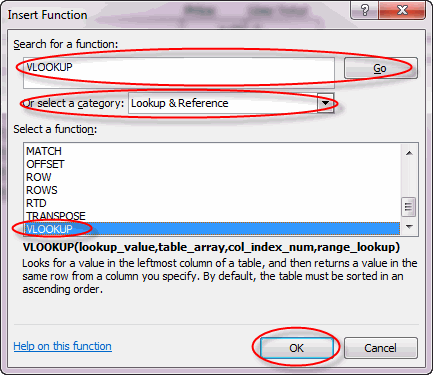# How to Use the VLOOKUP Function in Microsoft Excel

See Microsoft Excel: Tips and Tricks for similar articles.

The `VLOOKUP` function is used to pull a value from a list or table based on a corresponding value. For example, if you have a worksheet with a table showing employee names, hire date, and salary, you could use `VLOOKUP` in a separate worksheet to pull the hire date and salary for individual employees from the first worksheet. In this example, the employee name serves as a key, identifying which information from the first worksheet you wish to pull.

This feature works the same in all modern versions of Microsoft Excel: 2010, 2013, and 2016.

To use the `VLOOKUP` function, you need to know:

1. Lookup value. The value you will use to identify individual records in your table. The Lookup Value must be in the left-most column of your table.
2. Table array. The table that contains the data you will use `VLOOKUP` to retrieve. This table can be in another worksheet or even another workbook from the one in which you enter the `VLOOKUP` function.
3. Col index num. The Lookup Value is always in the left-most column of the Table Array (column #1, regardless of where in the worksheet the table is located). The next column to the right is column #2, then column #3, etc. The Col index num is simply the number of the column that contains the value you wish to retrieve.
4. Range lookup. Enter False if the Lookup Value must match exactly. If you enter True or leave blank, Excel will assume the table is sorted in ascending order and will select the best match. Note that if the table is not sorted in ascending order, Excel likely won't correctly find the best match.

To use the `VLOOKUP` function in Excel:

1. On the Formulas tab, in the Function Library group, click the Insert Function command.2. In the Insert Function dialog box:
1. Search on "VLOOKUP" or, in the Or select a category drop-down box, select Lookup & Reference.
2. Under Select a function, select VLOOKUP.
3. Click OK.3. In the Function Arguments dialog box:
1. Enter the Lookup_value or the cell in which it is located.
2. Enter the Table_array.
3. Enter the Col_index_num.
4. Enter the Range_lookup. If you leave this blank, Excel will treat this as if you entered True.
5. Click OK.